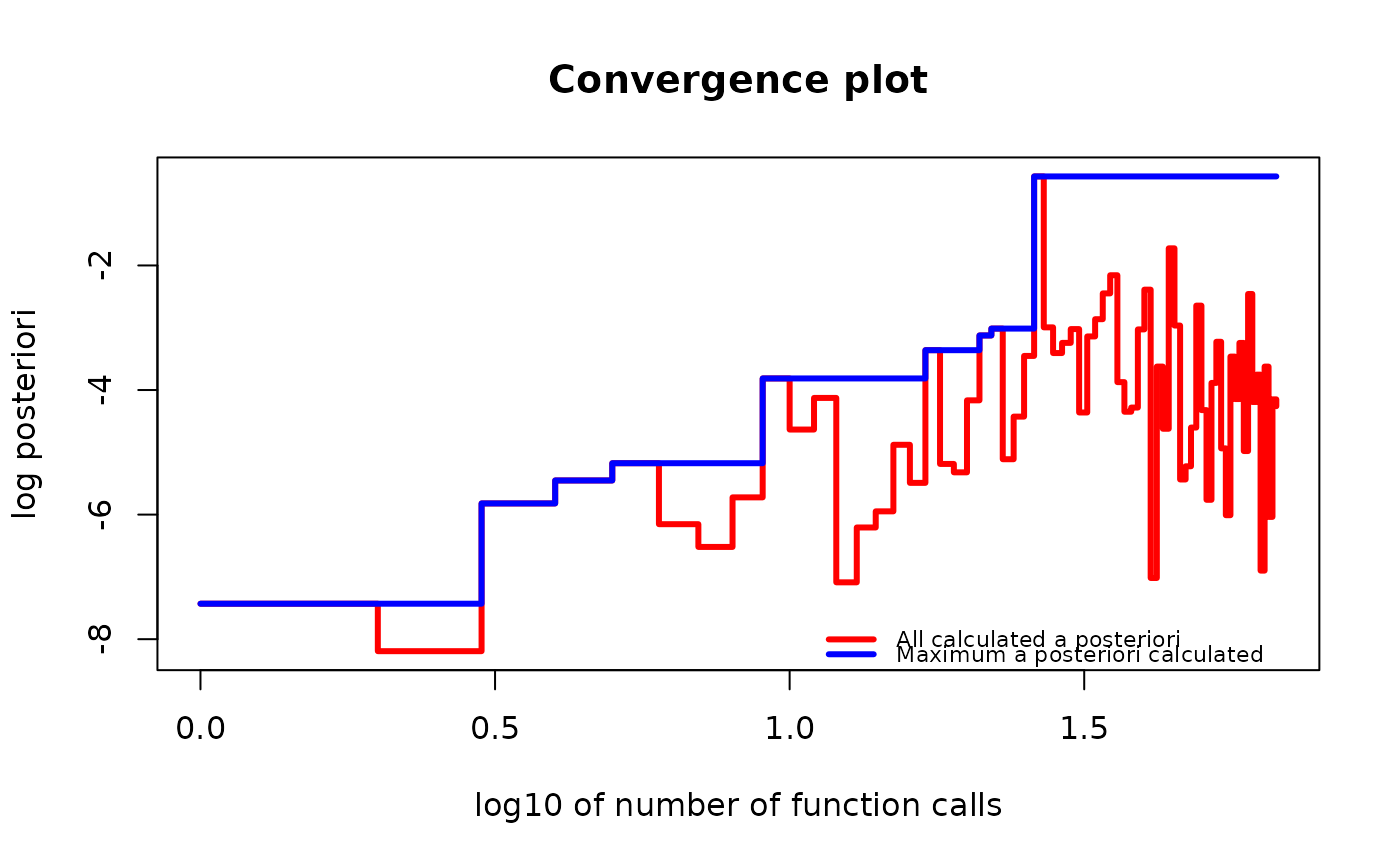Create a gips object. This object will contain initial data and all other information needed to find the most likely invariant permutation. It will not perform optimization. One must call the find_MAP() function to do it. See the examples below.

## Usage

gips(
S,
number_of_observations,
delta = 3,
D_matrix = NULL,
was_mean_estimated = TRUE,
perm = ""
)

new_gips(
list_of_gips_perm,
S,
number_of_observations,
delta,
D_matrix,
was_mean_estimated,
optimization_info
)

validate_gips(g)

## Arguments

S

A matrix; empirical covariance matrix. When Z is the observed data:

• if one does not know the theoretical mean and has to estimate it with the observed mean, use S = cov(Z), and leave parameter was_mean_estimated = TRUE as default;

• if one know the theoretical mean is 0, use S = (t(Z) %*% Z) / number_of_observations, and set parameter was_mean_estimated = FALSE.

number_of_observations

A number of data points that S is based on.

delta

A number, hyper-parameter of a Bayesian model. It has to be strictly bigger than 1. See the Hyperparameters section below.

D_matrix

Symmetric, positive-definite matrix of the same size as S. Hyper-parameter of a Bayesian model. When NULL, the (hopefully) reasonable one is derived from the data. For more details, see the Hyperparameters section below.

was_mean_estimated

A boolean.

• Set TRUE (default) when your S parameter is a result of a stats::cov() function.

• Set FALSE when your S parameter is a result of a (t(Z) %*% Z) / number_of_observations calculation.

perm

An optional permutation to be the base for the gips object. It can be of a gips_perm or a permutation class, or anything the function permutations::permutation() can handle. It can also be of a gips class, but it will be interpreted as the underlying gips_perm.

list_of_gips_perm

A list with a single element of a gips_perm class. The base object for the gips object.

optimization_info

For internal use only. NULL or the list with information about the optimization process.

g

Object to be checked whether it is a proper object of a gips class.

## Value

gips() returns an object of a gips class after the safety checks.

new_gips() returns an object of a gips class without the safety checks.

validate_gips() returns its argument unchanged. If the argument is not a proper element of a gips class, it produces an error.

## Functions

• new_gips(): Constructor. It is only intended for low-level use.

• validate_gips(): Validator. It is only intended for low-level use.

## Methods for a gips class

• summary.gips()

• plot.gips()

• print.gips()

• logLik.gips()

• AIC.gips()

• BIC.gips()

• as.character.gips()

## Hyperparameters

We encourage the user to try D_matrix = d * I, where I is an identity matrix of a size p x p and d > 0 for some different d. When d is small compared to the data (e.g., d=0.1 * mean(diag(S))), bigger structures will be found. When d is big compared to the data (e.g., d=100 * mean(diag(S))), the posterior distribution does not depend on the data.

Taking D_matrix = d * I is equivalent to setting S <- S / d.

The default for D_matrix is D_matrix = d * I, where d = mean(diag(S)), which is equivalent to modifying S so that the mean value on the diagonal is 1.

In the Bayesian model, the prior distribution for the covariance matrix is a generalized case of Wishart distribution.

For a brief introduction, see the Bayesian model selection section in vignette("Theory", package = "gips") or in its pkgdown page).

For analysis of the Hyperparameters influence, see Section 3.2. of "Learning permutation symmetries with gips in R" by gips developers Adam Chojecki, Paweł Morgen, and Bartosz Kołodziejek, available on arXiv:2307.00790.

• stats::cov() - The S parameter, as an empirical covariance matrix, is most of the time a result of the cov() function. For more information, see Wikipedia - Estimation of covariance matrices.

• find_MAP() - The function that finds the Maximum A Posteriori (MAP) Estimator for a given gips object.

• gips_perm() - The constructor of a gips_perm class. The gips_perm object is used as the base object for the gips object. To be more precise, the base object for gips is a one-element list of a gips_perm object.

## Examples

require("MASS") # for mvrnorm()

perm_size <- 5
mu <- runif(5, -10, 10) # Assume we don't know the mean
sigma_matrix <- matrix(
data = c(
1.0, 0.8, 0.6, 0.6, 0.8,
0.8, 1.0, 0.8, 0.6, 0.6,
0.6, 0.8, 1.0, 0.8, 0.6,
0.6, 0.6, 0.8, 1.0, 0.8,
0.8, 0.6, 0.6, 0.8, 1.0
),
nrow = perm_size, byrow = TRUE
) # sigma_matrix is a matrix invariant under permutation (1,2,3,4,5)
number_of_observations <- 13
Z <- MASS::mvrnorm(number_of_observations, mu = mu, Sigma = sigma_matrix)
S <- cov(Z) # Assume we have to estimate the mean

g <- gips(S, number_of_observations)

g_map <- find_MAP(g, show_progress_bar = FALSE, optimizer = "brute_force")
g_map
#> The permutation (1,3)(4,5):
#>  - was found after 67 posteriori calculations;
#>  - is 22.389 times more likely than the () permutation.

summary(g_map)
#> The optimized gips object.
#>
#> Permutation:
#>  (1,3)(4,5)
#>
#> Log_posteriori:
#>  -8.130075
#>
#> Times more likely than starting permutation:
#>  22.389
#>
#> The number of observations:
#>  13
#>
#> The mean in the S matrix was estimated.
#> Therefore, one degree of freedom was lost.
#> There are 12 degrees of freedom left.
#>
#> n0:
#>  4
#>
#> The number of observations is bigger than n0 for this permutation,
#> so the gips model based on the found permutation does exist.
#>
#> The number of free parameters in the covariance matrix:
#>  9
#>
#> BIC:
#>  111.5481
#>
#> AIC:
#>  106.4636
#>
#> --------------------------------------------------------------------------------
#> Optimization algorithm:
#>  brute_force
#>
#> The number of log_posteriori calls:
#>  67
#>
#> Optimization time:
#>  0.1594262 secs

if (require("graphics")) {
plot(g_map, type = "both", logarithmic_x = TRUE)
}